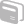# Activities

•• ##### Subject Area

• Other Subjects: Test Prep Resources: SAT Questions

• ##### Author9-12
College

15 Minutes

• ##### Device
•TI-Nspire™ CX CAS/CX II CAS
• ##### Software

TI-Nspire™ CX CAS

4.2

## SAT Math Made Easy - Trial

#### Activity Overview

In SAT Math Made Easy - Trial Edition, students will use TI-Nspire™ technology to explore common SAT math problems utilizing step-by-step processes.

#### Vocabulary

• Composition of 2 Functions
• Slope, Midpoint, Distance given 2 points
• Solve Exponential / Money Growth problems
• Geometric Sequences and Series
• Solve Side-Side-Side Triangles
• Count Integers over a range with certain properties (repeatable digits, divisible by digit, contains digit, etc )

In "SAT Made Easy- Trial Version", there are 7 different activities for students to explore.

To access the different activities within the TNS file, from the title screen, MENU >

• Algebra> (2) Solve a 2x2 System of Equations: Learn how to solve a 2x2 system of equation using the elimination method.
• Functions >(6) Composition of 2 Functions : Learn Step by Step how to compose two function and evaluate it.
• Points & Lines> (4) Slope, Midpoint, Distance :Learn Step by Step how to find Slope, Midpoint and Distance given 2 points.
• QuadraticFunctions > (1) Do the Quadratic Equation: Find Step by Step the Zeros of a Quadratic Function using the Quadratic Equation .
• Exponents & Logarithms > (4) Exponential Money Growth : Find Step by Step how the invested principal grows at given time.
• Sequences & Series > (4) Geometric Sequence and Series: Find Step by Step the terms and the sum of a geometric Sequence.
• Trigonometry > (5) SSS Triangle : Solve Step by Step a triangle given its 3 sides using the law of cosine
• Specials > (3) Count Integers with certain properties:Compute the Number of Integers with given properties such as repeatable digits, divisible by digit, contains digit.

Note that all items with a check-mark next to it are functional in this trial version, so there are more activities available in the file to preview then those listed above. For more information on purchasing the full version, visit SMARTSOFT.